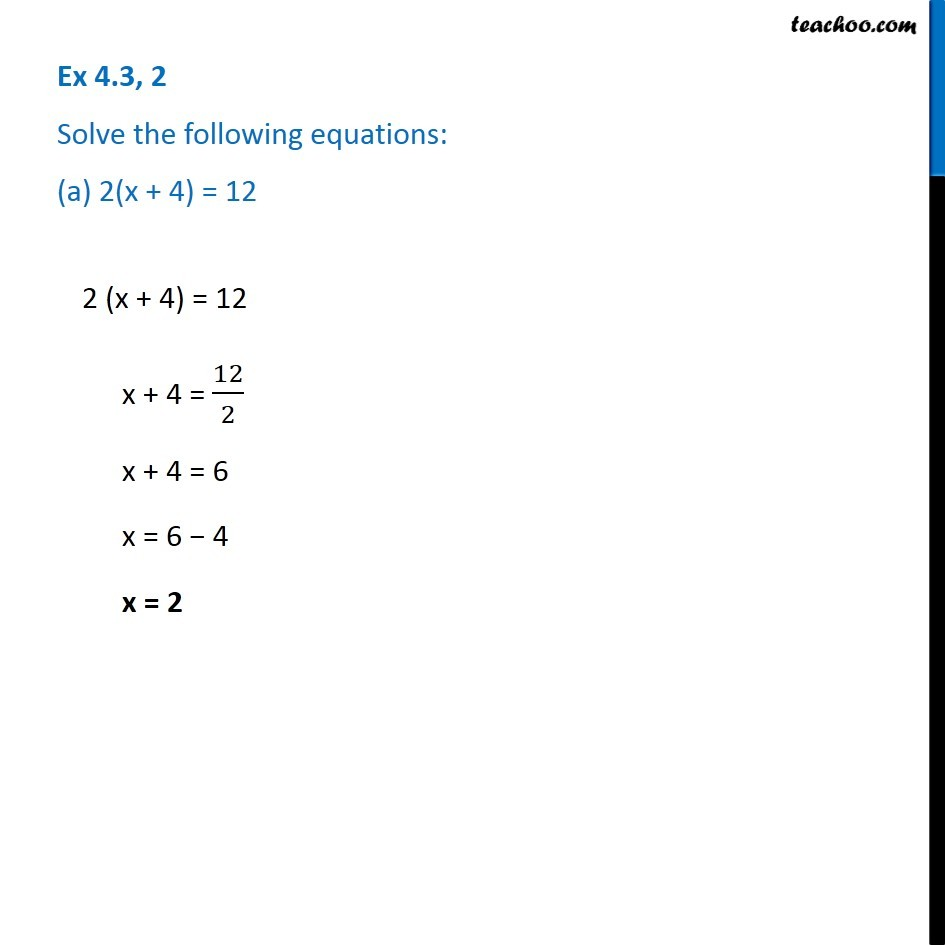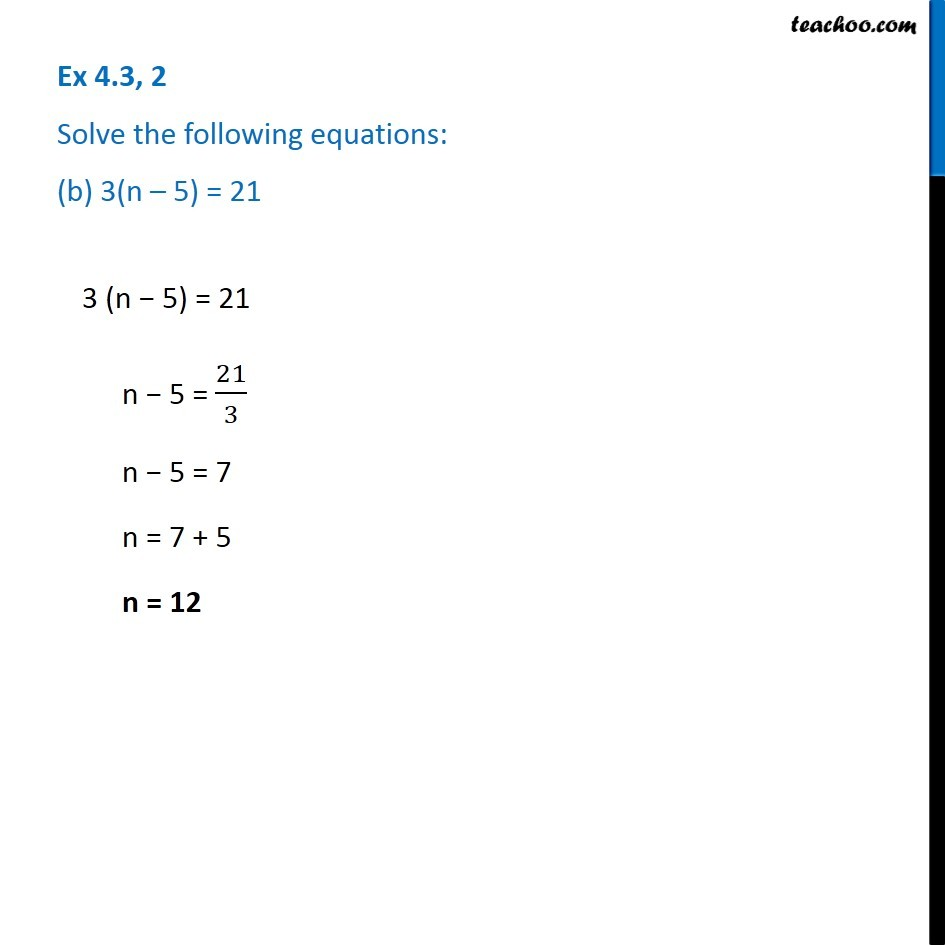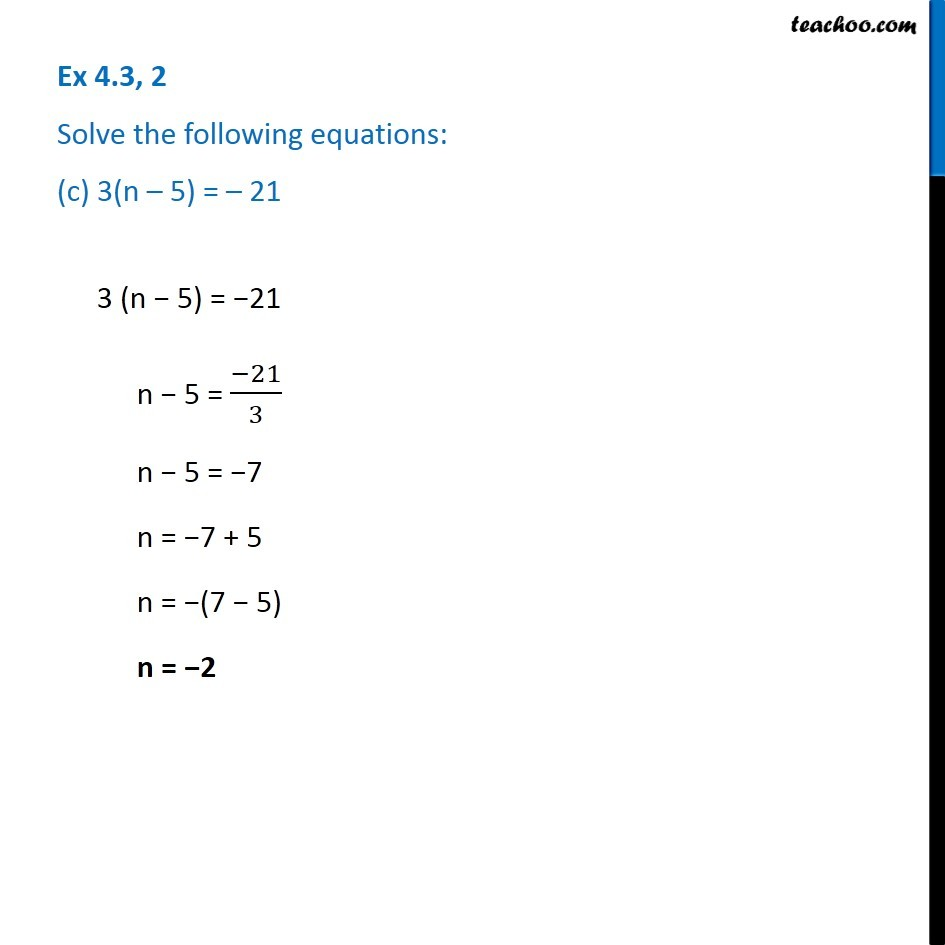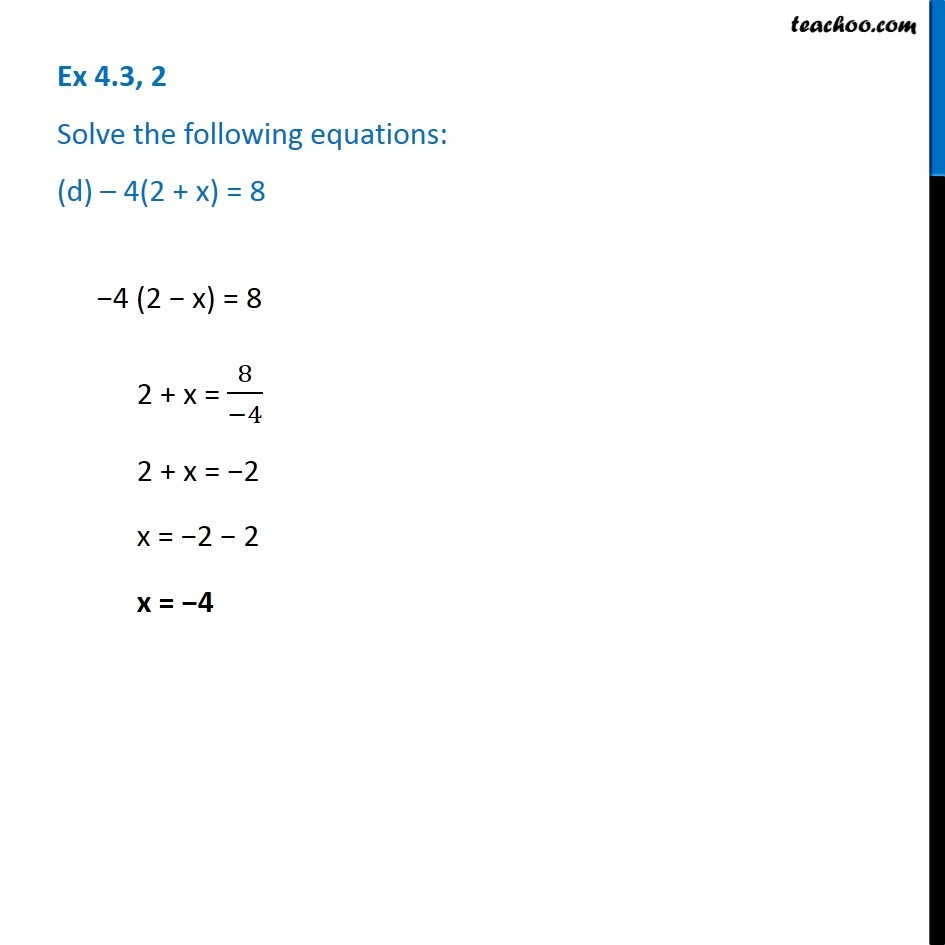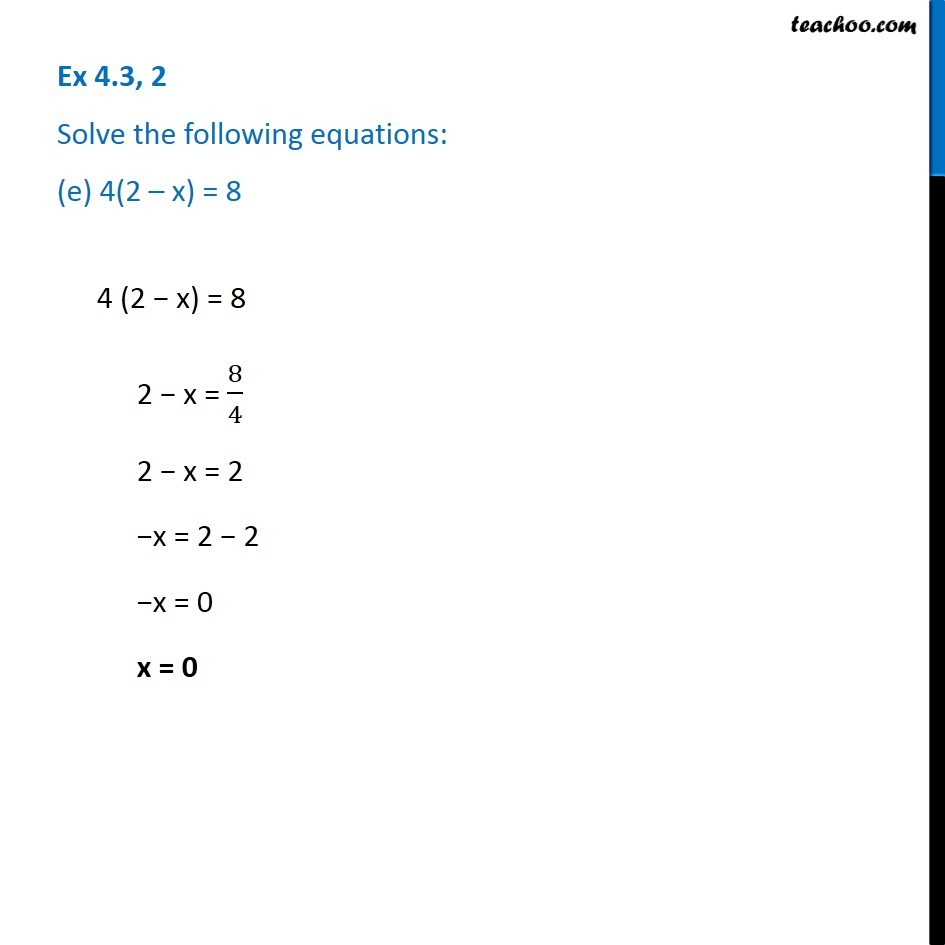1. Chapter 4 Class 7 Simple Equations
2. Concept wise
3. Solving Equations

Transcript

Ex 4.3, 2 Solve the following equations: (a) 2(x + 4) = 122 (x + 4) = 12 x + 4 = 12/2 x + 4 = 6 x = 6 − 4 x = 2 Ex 4.3, 2 Solve the following equations: (b) 3(n – 5) = 213 (n − 5) = 21 n − 5 = 21/3 n − 5 = 7 n = 7 + 5 n = 12 3 (n − 5) = 21 n − 5 = 21/3 n − 5 = 7 n = 7 + 5 n = 12 3 (n − 5) = −21 n − 5 = (−21)/3 n − 5 = −7 n = −7 + 5 n = −(7 − 5) n = −2 Ex 4.3, 2 Solve the following equations: (d) – 4(2 + x) = 8−4 (2 − x) = 8 2 + x = 8/(−4) 2 + x = −2 x = −2 − 2 x = −4 Ex 4.3, 2 Solve the following equations: (e) 4(2 – x) = 84 (2 − x) = 8 2 − x = 8/4 2 − x = 2 −x = 2 − 2 −x = 0 x = 0

Solving Equations